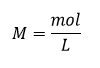# Problem: How many milliliters of a 0.1500-M solution of KOH will be required to titrate 40.00 mL of a 0.0656-M solution of H3PO4?H3PO4(aq) + 2KOH(aq) ⟶ K2HPO4(aq) + 2H2O(l)

###### FREE Expert Solution

Since the given reaction is already balanced, we can do a mole to mole comparison to calculate the number of moles of KOH to react with H3PO4 then calculate the volume of KOH solution needed.

Recall:79% (448 ratings)###### Problem Details

How many milliliters of a 0.1500-M solution of KOH will be required to titrate 40.00 mL of a 0.0656-M solution of H3PO4?

H3PO4(aq) + 2KOH(aq) ⟶ K2HPO4(aq) + 2H2O(l)